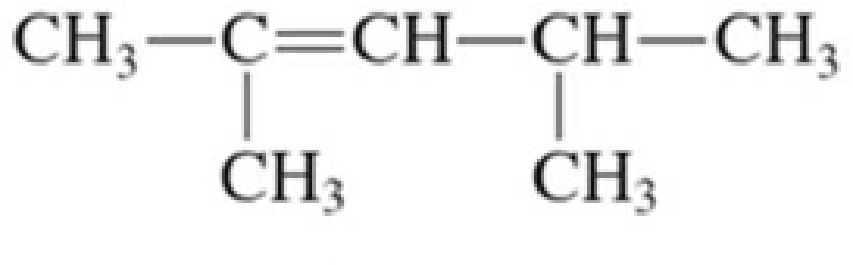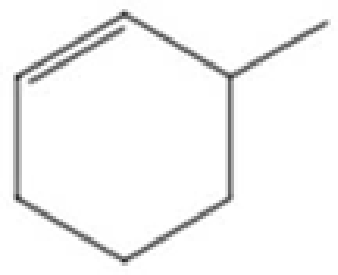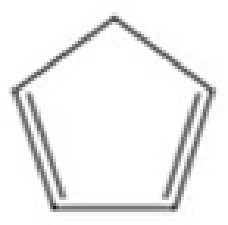Chapter 2, Problem 2.17EP### Organic And Biological Chemistry

7th Edition
STOKER + 1 other
ISBN: 9781305081079

#### Solutions

Chapter
Section### Organic And Biological Chemistry

7th Edition
STOKER + 1 other
ISBN: 9781305081079
Textbook Problem

# Assign an IUPAC name to each of the following unsaturated hydrocarbons.a. CH3—CH=CH—CH3b.c.d.(a)

Interpretation Introduction

Interpretation:

IUPAC name for the given unsaturated hydrocarbon has to be assigned.

Concept Introduction:

IUPAC nomenclature for alkene:  There are about eight rules to be followed in giving IUPAC name for alkene.

• The suffix –ane has to be replaced with the suffix –ene.  This is used to indicate the presence of double bond.
• The longest continuous chain of carbon atoms has to be chosen that contains both carbon atoms of the double bond.
• The parent carbon chain has to be numbered in a way so that the numbering begins at the end near to the double bond.  In case if the double bond is equidistant from both ends, then numbering has to be done from the end that is closer to substituents.
• The position of the double bond has to be given a single number which is lower‑numbered carbon atom that is present in the double bond.
• Suffixes like –diene, -triene, -tetrene, and so on are used when the compound contains more than one double bond.
• In case of cycloalkenes which do not have any substitution, the numbering is not needed to locate the double bond because the bond is assumed to be between the carbons 1 and 2.
• In case if substituents are present in cycloalkene, then the double‑bonded carbon atoms are numbered 1 and 2 in a direction where the substituent gets the lower number.
• If the cycloalkenes contain more than one double bond, then one double bond is assigned the numbers 1 and 2 followed by the other double bond so that the lowest number possible is given.
Explanation

Given compound is CH3CH=CHCH3.  The total number of carbon atom present in the given compound is four.  Hence, the parent alkane is butane.

As the given compound contains one double bond, the suffix –ane is replaced by –ene

(b)

Interpretation Introduction

Interpretation:

IUPAC name for the given unsaturated hydrocarbon has to be assigned.

Concept Introduction:

IUPAC nomenclature for alkene:  There are about eight rules to be followed in giving IUPAC name for alkene.

• The suffix –ane has to be replaced with the suffix –ene.  This is used to indicate the presence of double bond.
• The longest continuous chain of carbon atoms has to be chosen that contains both carbon atoms of the double bond.
• The parent carbon chain has to be numbered in a way so that the numbering begins at the end near to the double bond.  In case if the double bond is equidistant from both ends, then numbering has to be done from the end that is closer to substituents.
• The position of the double bond has to be given a single number which is lower‑numbered carbon atom that is present in the double bond.
• Suffixes like –diene, -triene, -tetrene, and so on are used when the compound contains more than one double bond.
• In case of cycloalkenes which do not have any substitution, the numbering is not needed to locate the double bond because the bond is assumed to be between the carbons 1 and 2.
• In case if substituents are present in cycloalkene, then the double‑bonded carbon atoms are numbered 1 and 2 in a direction where the substituent gets the lower number.
• If the cycloalkenes contain more than one double bond, then one double bond is assigned the numbers 1 and 2 followed by the other double bond so that the lowest number possible is given.

(c)

Interpretation Introduction

Interpretation:

IUPAC name for the given unsaturated hydrocarbon has to be assigned.

Concept Introduction:

IUPAC nomenclature for alkene:  There are about eight rules to be followed in giving IUPAC name for alkene.

• The suffix –ane has to be replaced with the suffix –ene.  This is used to indicate the presence of double bond.
• The longest continuous chain of carbon atoms has to be chosen that contains both carbon atoms of the double bond.
• The parent carbon chain has to be numbered in a way so that the numbering begins at the end near to the double bond.  In case if the double bond is equidistant from both ends, then numbering has to be done from the end that is closer to substituents.
• The position of the double bond has to be given a single number which is lower‑numbered carbon atom that is present in the double bond.
• Suffixes like –diene, -triene, -tetrene, and so on are used when the compound contains more than one double bond.
• In case of cycloalkenes which do not have any substitution, the numbering is not needed to locate the double bond because the bond is assumed to be between the carbons 1 and 2.
• In case if substituents are present in cycloalkene, then the double‑bonded carbon atoms are numbered 1 and 2 in a direction where the substituent gets the lower number.
• If the cycloalkenes contain more than one double bond, then one double bond is assigned the numbers 1 and 2 followed by the other double bond so that the lowest number possible is given.

(d)

Interpretation Introduction

Interpretation:

IUPAC name for the given unsaturated hydrocarbon has to be assigned.

Concept Introduction:

IUPAC nomenclature for alkene:  There are about eight rules to be followed in giving IUPAC name for alkenes.

• The suffix –ane has to be replaced with the suffix –ene.  This is used to indicate the presence of double bond.
• The longest continuous chain of carbon atoms has to be chosen that contains both carbon atoms of the double bond.
• The parent carbon chain has to be numbered in a way so that the numbering begins at the end near to the double bond.  In case if the double bond is equidistant from both ends, then numbering has to be done from the end that is closer to substituents.
• The position of the double bond has to be given a single number which is lower‑numbered carbon atom that is present in the double bond.
• Suffixes like –diene, -triene, -tetrene, and so on are used when the compound contains more than one double bond.
• In case of cycloalkenes which do not have any substitution, the numbering is not needed to locate the double bond because the bond is assumed to be between the carbons 1 and 2.
• In case if substituents are present in cycloalkene, then the double‑bonded carbon atoms are numbered 1 and 2 in a direction where the substituent gets the lower number.
• If the cycloalkenes contain more than one double bond, then one double bond is assigned the numbers 1 and 2 followed by the other double bond so that the lowest number possible is given.

### Still sussing out bartleby?

Check out a sample textbook solution.

See a sample solution

#### The Solution to Your Study Problems

Bartleby provides explanations to thousands of textbook problems written by our experts, many with advanced degrees!

Get Started